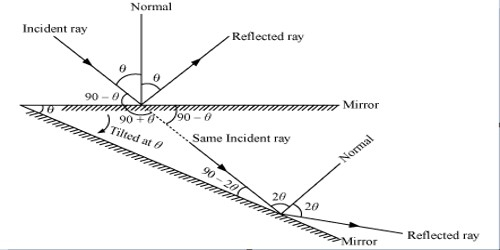# Explain Deviation of Light due to Rotation of a Mirror

A ray of light is the path along which the lights travel. Rays are observable. They travel along the straight line in a similar homogeneous medium and are represented by straight lines with arrowheads. The arrowhead indicates the path of light. Consider a plane-mirror and a fixed incident ray of light reflecting from the surface at an angle θ. Before the mirror has rotated, the angle of incidence is θ as is the angle of reflection.

Deviation of Light due to Rotation of a Mirror: Let consider al ray of light AO incident on a plane mirror XY at O. It is reflected along OB. Let α be the glancing angle with XY (Figure). We know that the angle of deviation COB = 2α.Suppose the mirror is rotated through an angle O to a position X’Y’.

The same incident ray AO is now reflected along OP. Here the glancing angle with X’Y’ is (α + O). Hence the new angle of deviation COP = 2 (α + θ). The reflected ray has thus been rotated through an angle BOP when the mirror is rotated through an angle θ.

Angle BOP = Angle COP – Angle COB

so, Angle BOP = 2 (α + θ) – 2α = 2θ

For the same incident ray, when the mirror is rotated through an angle, the reflected ray is rotated through twice the angle. If an object is positioned between two plane mirrors disposed at an angle θ, then the number of images formed is n = (360º/θ) – 1.

Characteristics of the Image Formed by a Plane Mirror

• Image shaped by a plane mirror is as far behind the mirror as the object is in front of it and it is always virtual.
• The smallest size of the mirror required to see the total image of the object is half the size of the object.
• If the mirror turns by an angle θ, the reflected ray turns through an angle 2θ.